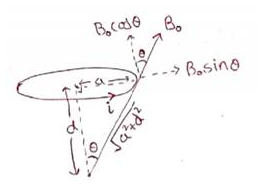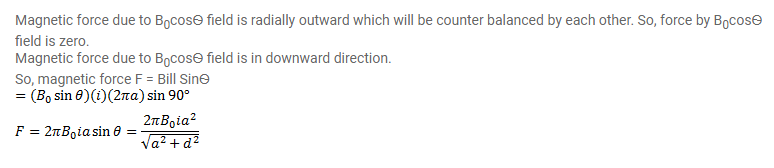# A hypothetical magnetic field existing in a region is given by

Question:

A hypothetical magnetic field existing in a region is given by $\vec{B}=B_{0} \vec{e}_{r}$, where $\vec{e}_{r}$ denotes the unit vector along the radial direction. A circular loop of radius a, carrying a current $i$, is placed with its plane parallel to the X-Y plane and the center at ( 0 , $0, d)$. Find the magnitude of the magnetic force acting on the loop.

Solution: